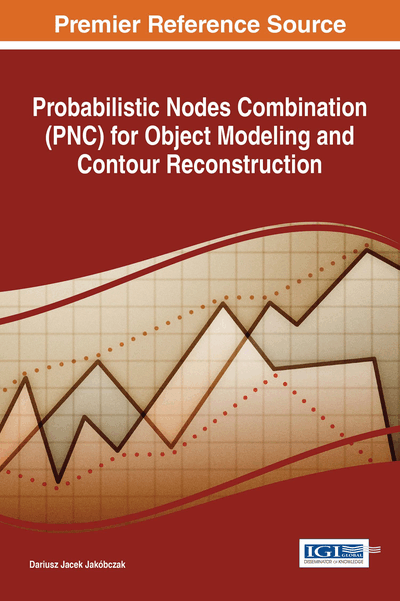# PNC in 3D Surface Modeling

DOI: 10.4018/978-1-5225-2531-8.ch005
Available
\$37.50
No Current Special Offers

## Abstract

The model of data can be built by choice of probability distribution function and nodes combination. PFC modeling via nodes combination and parameter ? as probability distribution function enables value anticipation in risk analysis and decision making. Two-dimensional curve is extrapolated and interpolated via nodes combination and different functions as discrete or continuous probability distribution functions: polynomial, sine, cosine, tangent, cotangent, logarithm, exponent, arc sin, arc cos, arc tan, arc cot or power function. The method of Probabilistic Features Combination (PFC) enables interpolation and modeling of high-dimensional data using features' combinations and different coefficients ? as modeling function. Functions for ? calculations are chosen individually at each data modeling and it is treated as N-dimensional probability distribution function: ? depends on initial requirements and features' specifications. PFC method leads to data interpolation as handwriting or signature identification and image retrieval via discrete set of feature vectors in N-dimensional feature space.
Chapter Preview
Top

## Background

Current methods apply mainly polynomial functions, for example Bernstein polynomials in Bezier curves, splines (Schumaker, 2007) and NURBS. But Bezier curves don’t represent the interpolation method and cannot be used for example in signature and handwriting modeling with characteristic points (nodes). Numerical methods (Collins, 2003) for data interpolation are based on polynomial or trigonometric functions (Chapra, 2012), for example Lagrange, Newton, Aitken and Hermite methods (Ralston & Rabinowitz, 2001). These methods have some weak sides and are not sufficient for curve interpolation in the situations when the curve cannot be build by polynomials or trigonometric functions (Zhang & Lu, 2004).

## Complete Chapter List

Search this Book:
Reset